Inverse of two matrices and verifying properties

Chapter 4 Class 12 Determinants
Concept wise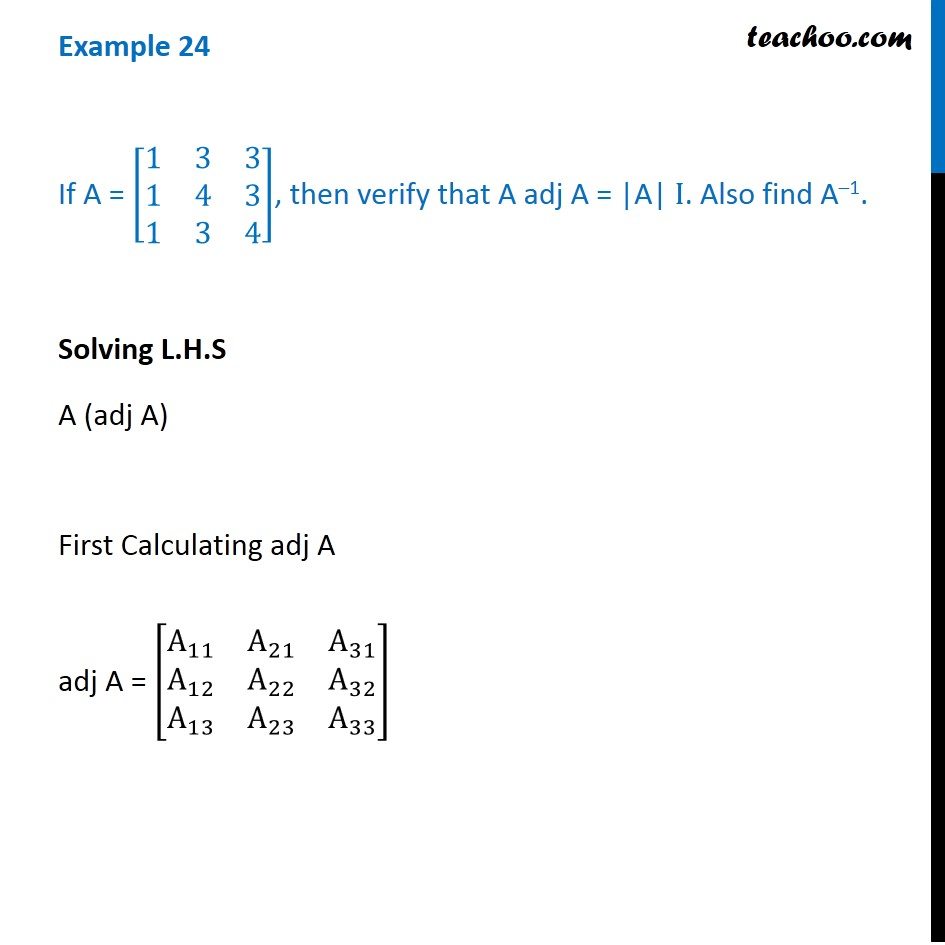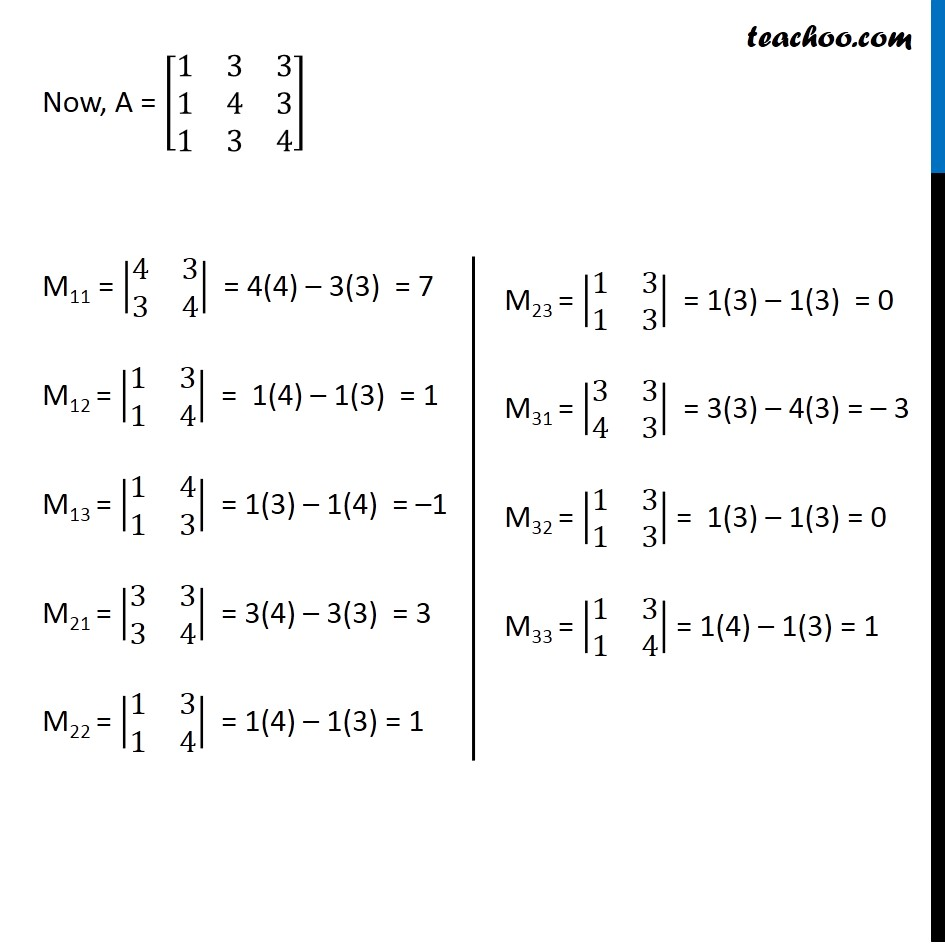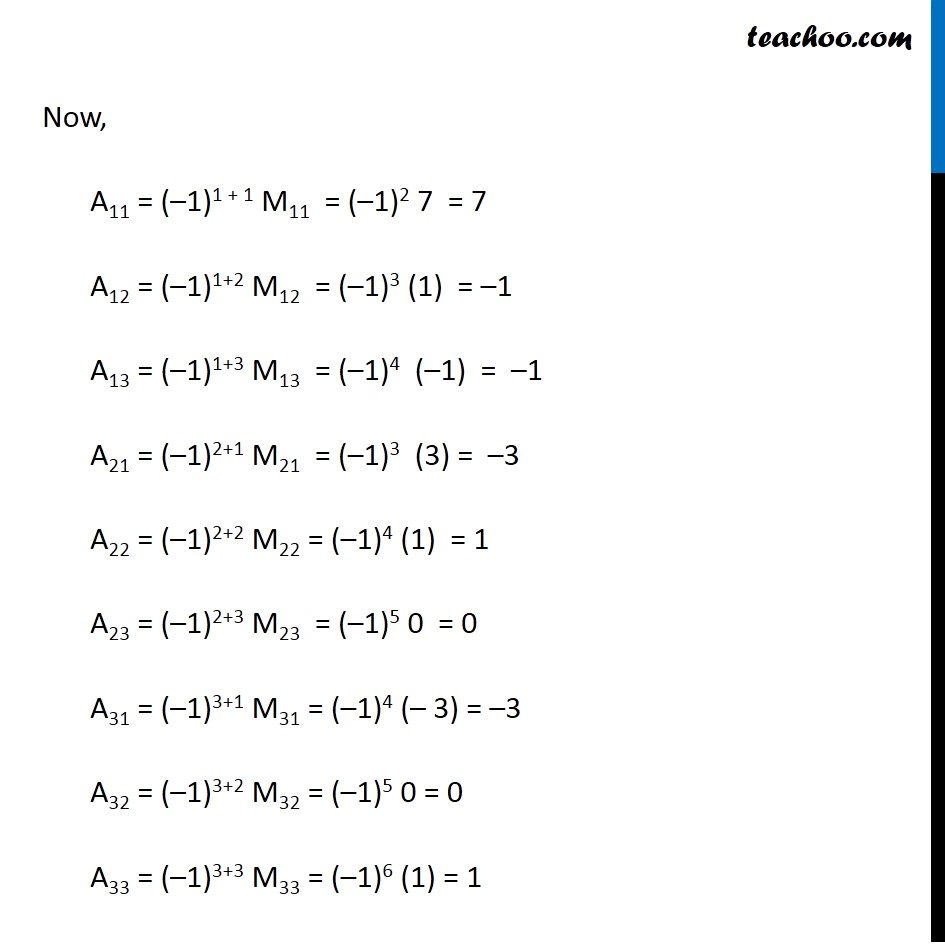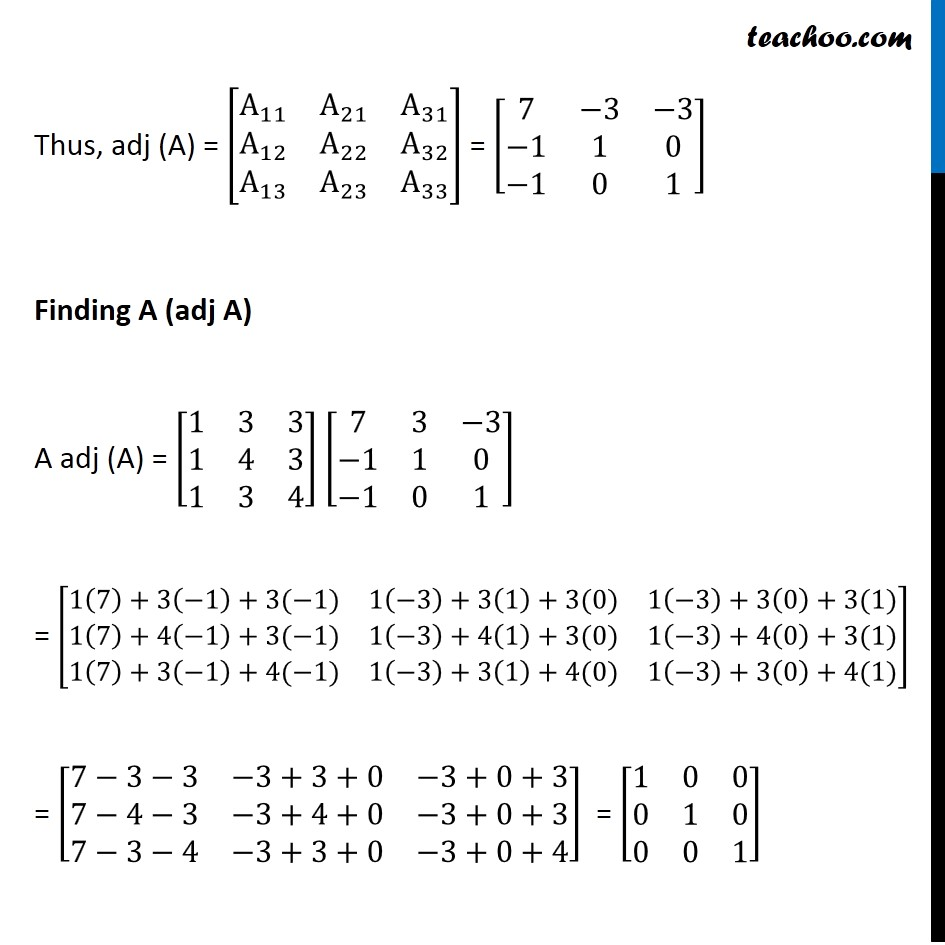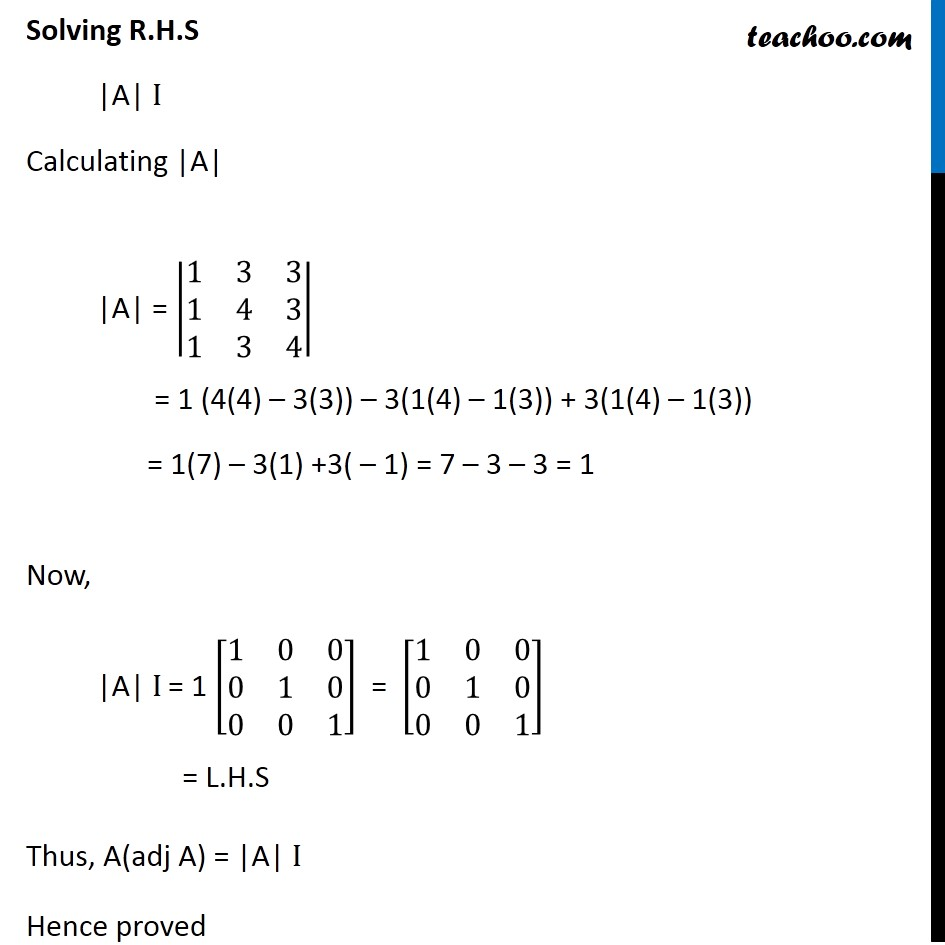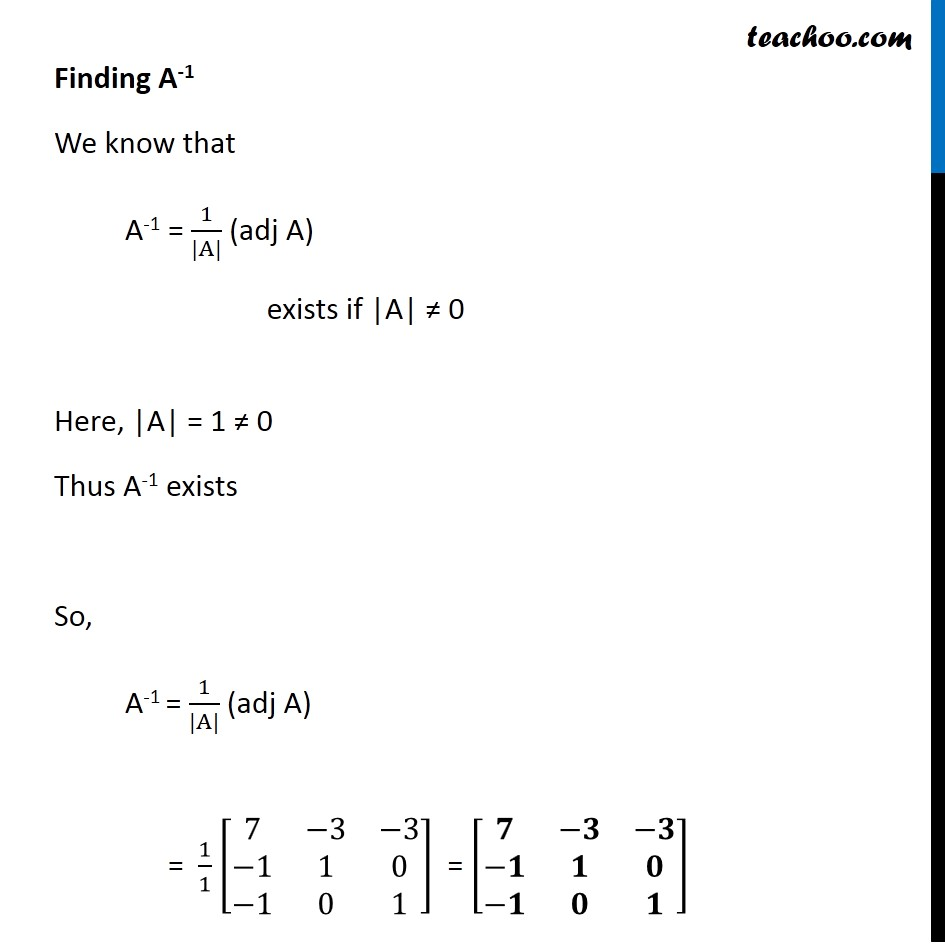Introducing your new favourite teacher - Teachoo Black, at only ₹83 per month

### Transcript

Example 24 If A = [■8(1&3&3@1&4&3@1&3&4)], then verify that A adj A = |A| I. Also find A–1. Solving L.H.S A (adj A) First Calculating adj A adj A = [■8(A_11&A_21&A_31@A_12&A_22&A_32@A_13&A_23&A_33 )] Now, A = [■8(1&3&3@1&4&3@1&3&4)] M11 = |■8(4&3@3&4)| = 4(4) – 3(3) = 7 M12 = |■8(1&3@1&4)| = 1(4) – 1(3) = 1 M13 = |■8(1&4@1&3)| = 1(3) – 1(4) = –1 M21 = |■8(3&3@3&4)| = 3(4) – 3(3) = 3 M22 = |■8(1&3@1&4)| = 1(4) – 1(3) = 1 M23 = |■8(1&3@1&3)| = 1(3) – 1(3) = 0 M31 = |■8(3&3@4&3)| = 3(3) – 4(3) = – 3 M32 = |■8(1&3@1&3)| = 1(3) – 1(3) = 0 M33 = |■8(1&3@1&4)| = 1(4) – 1(3) = 1 Now, A11 = (–1)1 + 1 M11 = (–1)2 7 = 7 A12 = (–1)1+2 M12 = (–1)3 (1) = –1 A13 = (–1)1+3 M13 = (–1)4 (–1) = –1 A21 = (–1)2+1 M21 = (–1)3 (3) = –3 A22 = (–1)2+2 M22 = (–1)4 (1) = 1 A23 = (–1)2+3 M23 = (–1)5 0 = 0 A31 = (–1)3+1 M31 = (–1)4 (– 3) = –3 A32 = (–1)3+2 M32 = (–1)5 0 = 0 A33 = (–1)3+3 M33 = (–1)6 (1) = 1 Thus, adj (A) = [■8(A_11&A_21&A_31@A_12&A_22&A_32@A_13&A_23&A_33 )] = [■8(7&−3&−3@−1&1&0@−1&0&1)] Finding A (adj A) A adj (A) = [■8(1&3&3@1&4&3@1&3&4)] [■8(7&3&−3@−1&1&0@−1&0&1)] = [■8(1(7)+3(⤶7−1)+3(−1)&1(−3)+3(1)+3(0)&1(−3)+3(0)+3(1)@1(7)+4(⤶7−1)+3(−1)&1(−3)+4(1)+3(0)&1(−3)+4(0)+3(1)@1(7)+3(⤶7−1)+4(−1)&1(−3)+3(1)+4(0)&1(−3)+3(0)+4(1))] = [■8(7−3−3&−3+3+0&−3+0+3@7−4−3&−3+4+0&−3+0+3@7−3−4&−3+3+0&−3+0+4)] = [■8(1&0&0@0&1&0@0&0&1)] Solving R.H.S |A| I Calculating |A| |A| = |■8(1&3&3@1&4&3@1&3&4)| = 1 (4(4) – 3(3)) – 3(1(4) – 1(3)) + 3(1(4) – 1(3)) = 1(7) – 3(1) +3( – 1) = 7 – 3 – 3 = 1 Now, |A| I = 1 [■8(1&0&0@0&1&0@0&0&1)] = [■8(1&0&0@0&1&0@0&0&1)] = L.H.S Thus, A(adj A) = |A| I Hence proved Finding A-1 We know that A-1 = 1/(|A|) (adj A) exists if |A| ≠ 0 Here, |A| = 1 ≠ 0 Thus A-1 exists So, A-1 = 1/(|A|) (adj A) = 1/1 [■8(7&−3&−3@−1&1&0@−1&0&1)] = [■8(𝟕&−𝟑&−𝟑@−𝟏&𝟏&𝟎@−𝟏&𝟎&𝟏)]